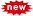### USER'S CHOICE POSTEarn upto Rs. 9,000 pm checking Emails. Join now! And get Rs. 99 Instantly!

ALL THE INFORMATION GIVEN IN THIS SITE IS ONLY FOR THE LEARNING PURPOSE. WE SHOULD ALWAYS USE THE GENUINE SOFTWARE! USE OF ANY INFORMATION GIVEN HERE IS SOLELY USERS' RESPONSIBILITY!

# SUM OF THE SERIES

Filled under: ,

SUM OF THE SERIES
13+23+33+43+……………+N3

#include<stdio.h>         //x=cube of each term(1+2+3+4+.....+n)
#include<conio.h>
void main()
{
int n,i,sum=0;
clrscr();
printf("Enter the nth term of series::");
scanf("%d",&n);
for(i=1;i<=n;i++)
{
sum=sum+(i*i*i);
}
printf("Value of x is %d",sum);
printf("Thank you!");
getch();
}

OUTPUT:
Enter the nth term of series: 3
value of x is 32
Thank you!